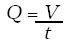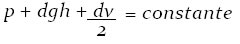Mechanics

# Hydrodynamics

Hydrodynamics has practical application in water supply systems, land irrigation, among others.

Hydrodynamics is responsible for studying the movement of fluids. Its practical application takes place in water supply systems, land irrigation, among others.

We will cover the basics of hydrodynamics.

Types and Flow:

Stationary Flow – also known as laminar, is obtained when the flow velocity is small, that is, when the flow velocity is the same at all points. Ex.: the water of a calm river, air flow and gases. Non -stationary or turbulent

flow is when the fluid’s velocity varies over time. Eg: waterfalls due to rocks and other existing obstacles.

The size of the tubes (diameter) and the viscosity of the fluid greatly influence the flow of fluids through the tubes, because, with the viscosity, forces of relative movement appear between the layers of the fluid, which causes the dissipation of mechanical energy.

Flow
It is defined as the ratio between volume and time.Q = flow rate
V = volume of fluid
t = time
Its SI unit is m³/s.

Continuity Equation
Determined by Castelli, a disciple of Galileo, says that the smaller the section, the greater the speed with which the fluid flows.

Pressure Velocity
The velocity of the fluid, when passing from a larger area to a smaller one, increases, because the pressure of the fluid in the wide part is greater than in the narrow part. This definition is also based on the continuity equation.

Bernouli
‘s equation Also called the fundamental equation of hydrodynamics, it was developed based on studies focused on the fluid flow energy.Where:
p = pressure (potential energy per unit volume)
dgh = hydrostatic pressure (gravitational potential energy per unit volume)
dv/2 = dynamic pressure (kinetic energy per unit volume)